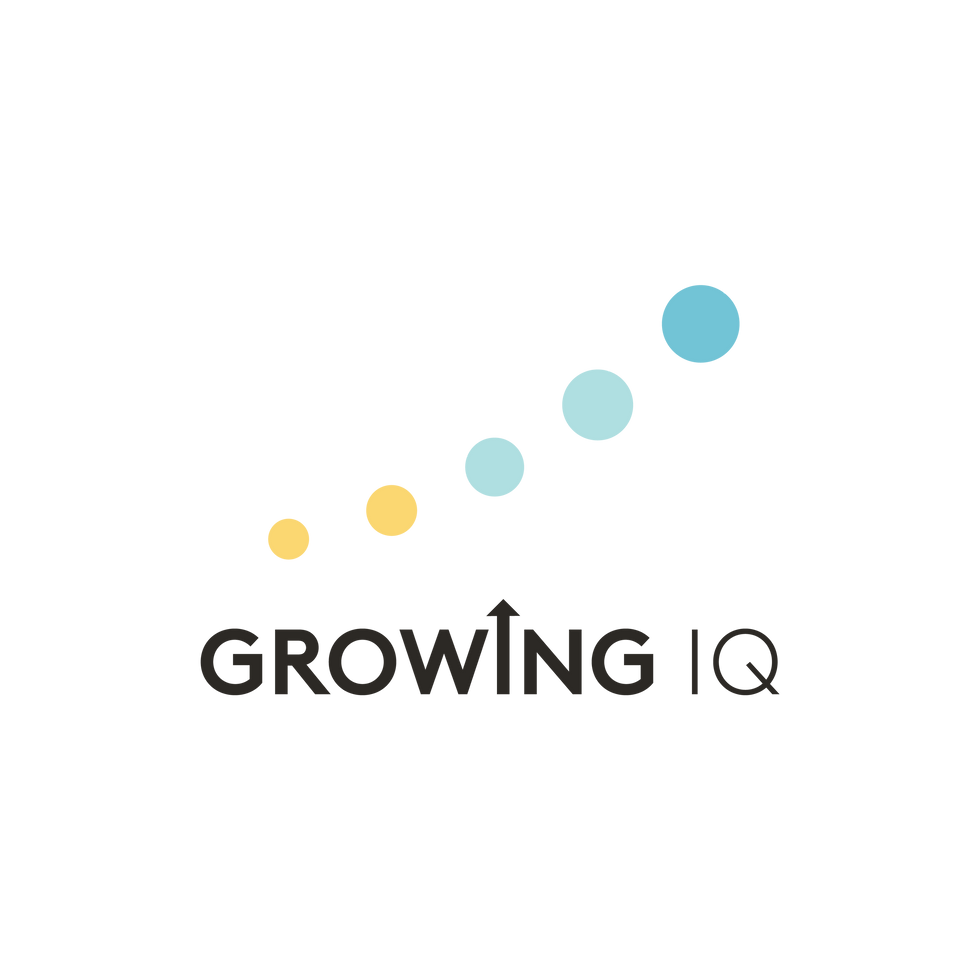top of page

A new way to approach Computer Science## Algorithmic Thinking

Coding Logic introduces students to enhanced problem-solving techniques by teaching them how to design and write out step-by-step algorithms. Algorithms the students create can be given to an information processing agent (person or computer) to solve problems or perform a task. From input to output, students will work together in classes to understand how algorithms are put together to solve various real-world problems using technology.## Decomposition

Coding Logic encourages students to use decomposition on problems, the process of breaking down a complicated problem into smaller, easier-to-solve problems. Decomposing problems helps with understanding patterns in problems/solutions, testing algorithms, and ﬁnding errors in algorithms. Having a problem broken down into simple less complicated tasks is extremely useful in making algorithms because the instructions technology uses are very simple and speciﬁc.

## Abstraction

Coding Logic promotes students to simplify problems by abstracting unnecessary details to help with understanding how to solve problems using an algorithmic process. Understanding how to use abstractions allows students to identify the important aspects of problems and not worry about unnecessary or unimportant details.## Generalization and Pattern Recognition

Generalization and pattern recognition enable students to reuse previous solutions and identify common patterns found in problem-solving. We can take an algorithm that we used to solve a speciﬁc problem and adapt it to solve a whole class of similar problems. An example of this is having to sort data, often times data must be sorted to accomplish a task. Whether someone is trying to sort information for a google search or sort a card collection, different types of data must be sorted but the algorithm for sorting the data can be the same.## Data Representation

Throughout the course, students are shown that data can be represented in many forms and practice using multiple encoding schemes (Base 10, Binary, Hexadecimal, Secret Codes, ASCII, etc.). Students get to explore these various ways of representing data and how those representations are interrelated. Students are shown how the decimal, binary, hexadecimal, and other number systems are designed and get a general understanding of how numeration systems are used.## Logic and Evaluation

Being a proﬁcient programmer relies heavily on the ability to use logical reasoning to make sense of problems, identify/collect data from programs, and evaluate solutions.  Logical reasoning allows one to use pre-existing knowledge to decide on a direction to approach problems and check information.## Interested in learning Coding Logic?

Currently, students interested in enrolling in Coding Logic must either have completed Level 5 of Growing IQ, show proficiency in the concepts addressed in Level 5, or be in 6th-8th grade.# Algorithmic Thinking# Decomposition# Abstraction# Generalization & Pattern Recognition# Data Representation# Logic & Evaluation

There are many skills and definitions that computational thinking encompasses,
for Coding Logic we use the following six computation thinking skills to help describe connections to lessons.

Curriculum

We do not view the ability to program as an isolated skill set. Coding skills incorporate computational thinking, computer science, mathematics, and numerous other subjects into the development process.

Our course helps students express their ideas in a specific form that a machine can understand and execute. Coding Logic's curriculum and teaching methodology of unplugged and plugged-in lessons expose students to the types of questions and problems that programmers often must deal with, as well as encourages students to use computational thinking skills.

1 hour per week

3 levels in totalExperience the power of play

2.5 - 6 years old

Math Music Motion exposes your child to mathematical concepts through the creative infusion of music and motion.

2 hours per week

6 levels in totalDerive & Discover

4 years old to 6th grade

Growing IQ develops your child's mindset through the discovery and exploration of math concepts, emphasizing critical thinking.

2 hours per week

2 levels in totalTackling problems logically

Coding Logic bridges math, computational thinking, and computer science engineering to prepare your child for the technological world we live in today.

Computational Thinking Skills

Computational thinking is the key concept taught in Coding Logic. Formally, computational thinking is a set of problem-solving methods that involve expressing problems and their solutions in ways that a computer could execute.The unplugged teaching methodology approaches teaching computational thinking, computer science, and coding without the use of any digital tech-nology. Students are given opportunities to look at problems in a new light and tackle them in ways that result in simple yet effective solutions. The use of code or coding skills is secondary to the logical instincts that give your child the ability to approach and unwind a problem. Once the underlying logic is better understood, transitioning to programming these solutions becomes a breeze. Our mission is to have students leave with the logical instincts that give them the ability to look at various problems and decide how to tackle them effectively.

Connecting computational thinking to codingDescribe the problem at hand

Identify the important details and ignore the unnecessary ones

Breaking a problem into smaller tasks that can be solved logically

Represent data in various forms that are useful for the problem at hand

Use instructions to solve each step and organize instructions into an algorithm

Evaluate the process used to solve the problem

Coding Logic gives students a foundation in computational thinking by teaching them how to:There are many skills and definitions that computational thinking encompasses, for Coding Logic we use the following six computation thinking skills to help describe connections to lessons.

bottom of page